2023
06-03

# python 算法教程（Python 插值算法）•线性插值
•双线性插值
•双三次插值bicubic interpolation

2. 插值算法原理和实现
2.1 线性插值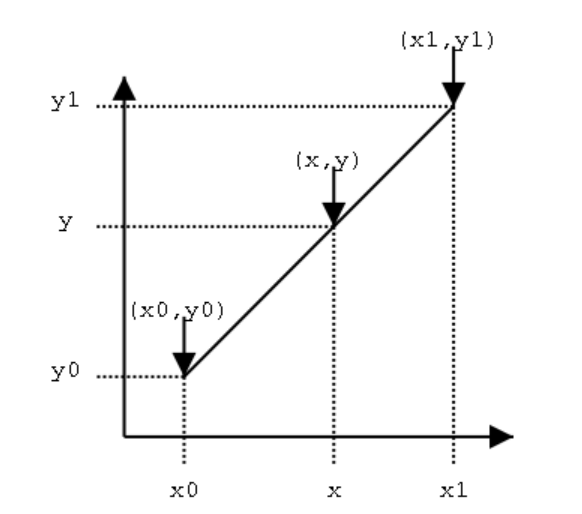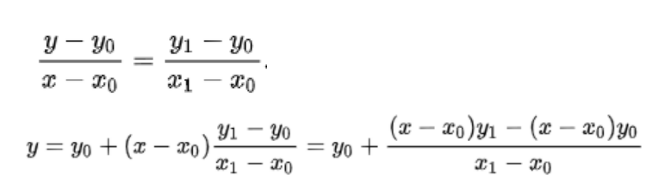import os
import numpy as np
from sklearn.linear_model import LinearRegression
def linear_interpolation(data, inter_num=4):
clf = LinearRegression()
X = np.array([, [inter_num+2]])
y = data
clf.fit(X, y)
inter_values = clf.predict(np.array([ [i+2] for i in range(inter_num)]))
return inter_values
data = [, ]
linear_interpolation(data, 1)
# array([[15.]])
def manual_linear_interpolation(x0, y0, x1, y1, x):
return y0+(x-x0)*(y1-y0)/(x1-x0)
manual_linear_interpolation(1, 10, 3, 20, 2)
# 15.0

2.2 双线性插值

•先插值生成(x, y1)(x, y0)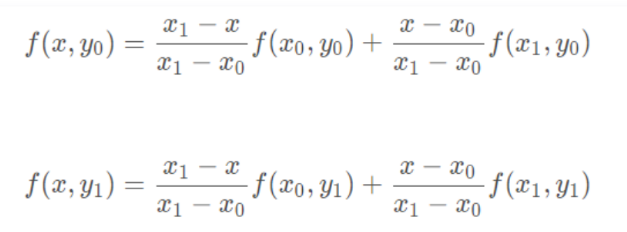•在插值生成(x, y)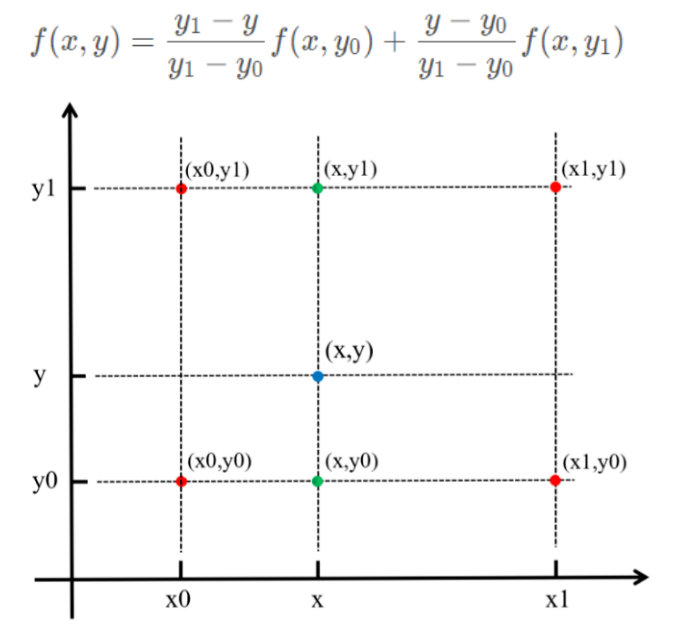import cv2
lena_x2 = cv2.resize(lean_img, (0, 0), fx=2, fy=2, interpolation=cv2.INTER_LINEAR)
2.3 双三次插值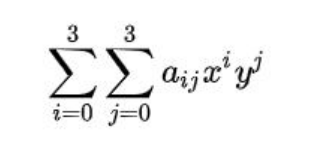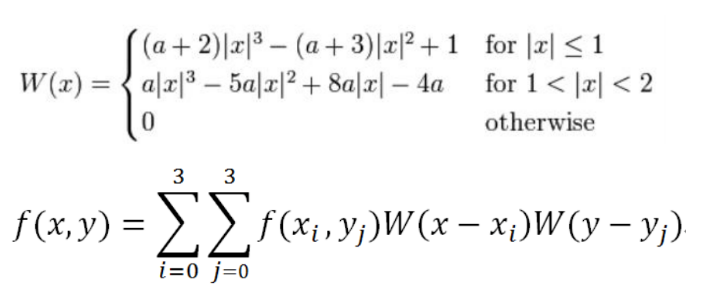import cv2